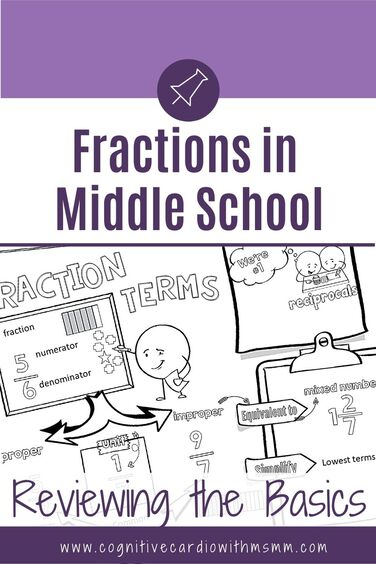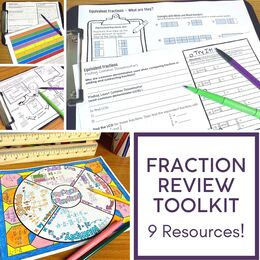# Fractions and Fraction Operations for Upper Elementary and Middle School Math

An Index of Fraction Content PostsAre you teaching fraction operations in middle school (or reteaching fraction concepts when they pop up in middle school?)
Are you always looking for fraction content or fraction teaching tips?
I’m here to help!
I’ve decided to do something I’ve never done on my blog:

• Since I have so many fraction-related posts for upper elementary and middle school math, I’ve added a couple new ‘summary’ posts as a kind of an index.
• One post (this one) will include the posts about teaching fraction concepts, and the other will summarize the posts about fraction games and activities

​So, let’s get to the fraction content posts.First, and newest, are the series of foundational fraction posts.

• Many of these posts include a free download or two, which can be accessed by registering for the free Fraction Review Toolkit.

The posts in the fraction series include:
1) Teaching Fractions in Middle School: Reviewing the Basics

• In this post we talk about the need that always seems to pop up – the need to review certain fraction concepts before moving on to your specific fraction curriculum.
• We review the meanings of fraction terms, how fractions are represented, and a few ways to weave fractions into class even when you aren’t teaching fraction concepts.

• This post addresses the progression of fraction skills through the elementary grades, up to 5th grade (and beyond).
• I share a few examples of using fraction models when adding and subtracting fractions and explore a combination of number lines & fraction strips.

• As the title indicates, this post focuses on using benchmarks, explores the 4th grade benchmark standard and includes examples of how to use estimation when adding fractions.

• Equivalent fractions are used in many situations, and we look at several of these in this post.
• We look at three ways to find common denominators in order to create equivalent fractions, and we explore representations of equivalent fractions.

## Fraction Operations in Middle School Content

In addition to the fraction series, I’ve written a few other fraction-related posts over the past several years.

Using the Ladder Method to Find LCD

• This one is a favorite of mine, because it includes the ladder method. It outlines the steps for finding the least common denominator and explains how this method has been helpful to students.

Fraction Division…Another Way

• This post is dedicated to teaching fraction division using the common denominator method, why this method works, and how my 6th grade students respond to the method.

Teaching Multiplication of Fractions and Mixed Numbers

• Multiplying mixed numbers can be tricky! Breaking it down with students can be so much fun.
• This post shares a common mistake students make, explains the numerical ‘breakdown’ of a problem and shows the breakdown using different representations.

Why NOT the Butterfly Method When Adding and Subtracting Fractions?

• I’ve had some pretty interesting experiences with the butterfly method in 6th grade math. This post share how I learned about this method and how my students used it.
Want even more (there’s so much more!) fraction and fraction operation content and materials all in one place?
Check out the program,

## EllieWelcome to Cognitive Cardio Math! I’m Ellie, a wife, mom, grandma, and dog ‘mom,’ and I’ve spent just about my whole life in school! With nearly 30 years in education, I’ve taught:

• All subject areas in 4th and 5th grades
• Math, ELA, and science in 6th grade (middle school)

I’ve been creating resources for teachers since 2012 and have worked in the elearning industry for about five years as well!

If you’re looking for ideas and resources to help you teach math (and a little ELA), I can help you out!